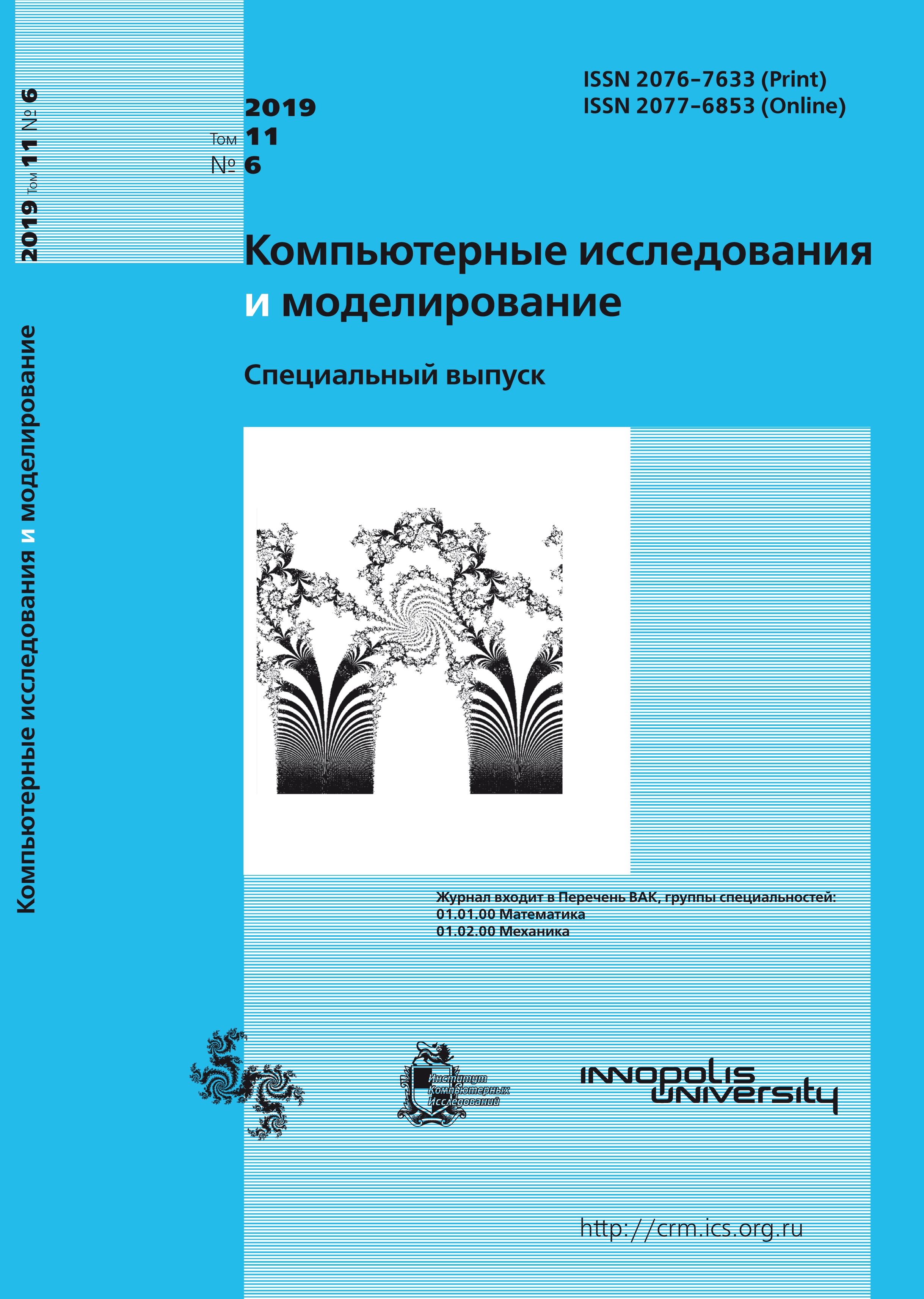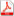Issue 6, 2019 Vol. 11

# All issues

Numerical Solution of Linear and Higher-order Delay Differential Equations using the Coded Differential Transform Methodpdf (138K)

The aim of the paper is to obtain a numerical solution for linear and higher-order delay differential equations (DDEs) using the coded differential transform method (CDTM). The CDTM is developed and applied to delay problems to show the efficiency of the proposed method. The coded differential transform method is a combination of the differential transform method and Mathematica software. We construct recursive relations for a few delay problems, which results in simultaneous equations, and solve them to obtain various series solution terms using the coded differential transform method. The numerical solution obtained by CDTM is compared with an exact solution. Numerical results and error analysis are presented for delay differential equations to show that the proposed method is suitable for solving delay differential equations. It is established that the delay differential equations under discussion are solvable in a specific domain. The error between the CDTM solution and the exact solution becomes very small if more terms are included in the series solution. The coded differential transform method reduces complex calculations, avoids discretization, linearization, and saves calculation time. In addition, it is easy to implement and robust. Error analysis shows that CDTM is consistent and converges fast. We obtain more accurate results using the coded differential transform method as compared to other methods.

Keywords: delay differential equations, coded differential transform method, numerical solution, mathematica
Citation in English: Methi G., Kumar A. Numerical Solution of Linear and Higher-order Delay Differential Equations using the Coded Differential Transform Method // Computer Research and Modeling, 2019, vol. 11, no. 6, pp. 1091-1099
Citation in English: Methi G., Kumar A. Numerical Solution of Linear and Higher-order Delay Differential Equations using the Coded Differential Transform Method // Computer Research and Modeling, 2019, vol. 11, no. 6, pp. 1091-1099
DOI: 10.20537/2076-7633-2019-11-6-1091-1099

Full-text version of the journal is also available on the web site of the scientific electronic library eLIBRARY.RU

The journal is included in the Russian Science Citation Index

The journal is included in the List of Russian peer-reviewed journals publishing the main research results of PhD and doctoral dissertations.

International Interdisciplinary Conference "Mathematics. Computing. Education"

The journal is included in the RSCI

Indexed in Scopus